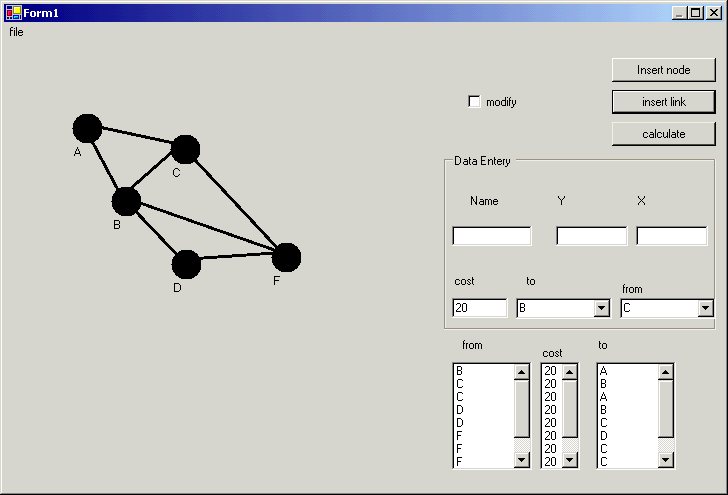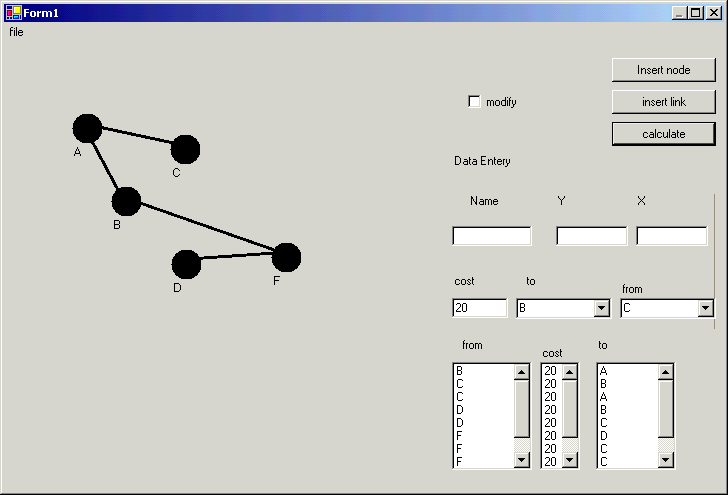# Implementing Prim's Algorithm using GDI+

Introduction:

This is a graphical implementation for Prim's algorithm. Prim's algorithm is an algorithm used in the graphics development to calculate the shortest route in a network when an object is moving from a source node to the destination node. For example, if a network has many nodes called A, B, C, D, and so on and every node has links to one another and there is a cost associated with the distance between two nodes. If we want to calculate the best way to move from point A to point D, we must have the minimum cost.

We implement this algorithm to choose any node randomly, say A and get the minimum link for it, for example this link to C. Then we have nodes A, and C and try to find the least cost link for both and so on. This application has two classes node and link.

How to use this program?

Simply type any name in the name textbox then press a click on the screen and so on to insert nodes as you like then choose from the combo boxes any two nodes, and type the distance then press insert link button.

If you want to modify the drag using drag and drop. Just check the modify check box and drag the graph.

Here is a graph with some nodes.Now here is the output after clicking the Calculate button.